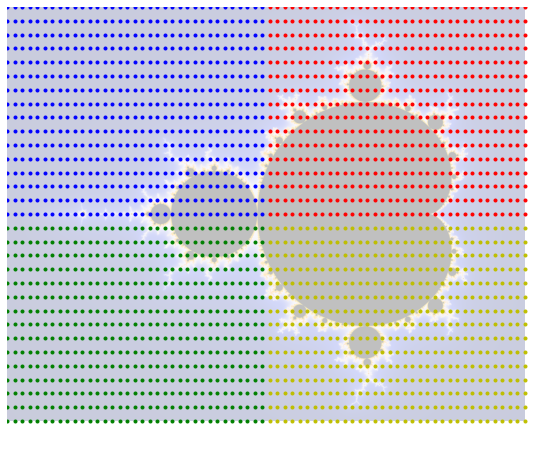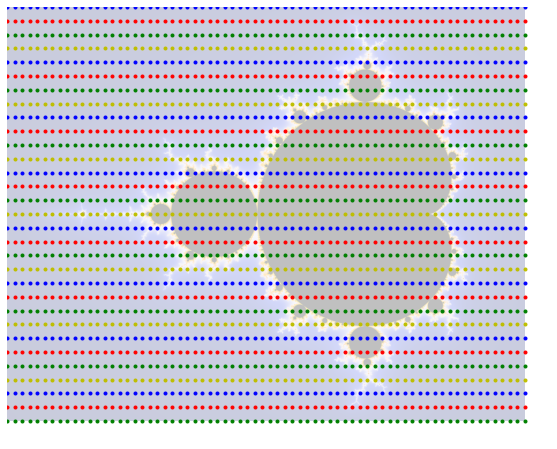# Implementing the Mandelbrot Set on DE1-SoC¶

#### V. Hunter Adams (vha3@cornell.edu)¶

In :
HTML('''<script>
code_show=true;
function code_toggle() {
if (code_show){
$('div.input').hide(); } else {$('div.input').show();
}
code_show = !code_show
}

### Parallelization and load balancing¶

To render the Mandelbrot Set as quickly as possible, you will want to be running the iteration on as many pixels simultaneously as you can with the hardware available on the FPGA. Think carefully about load balancing among your parallel solvers! Which of the below options best balances the load among four parallel solvers?

In :
x = numpy.linspace(-2, 1, 70)
y = numpy.linspace(-1, 1, 31)
X, Y = numpy.meshgrid(x, y)
plt.figure(2, figsize=(12, 8))
implot = plt.imshow(im, zorder=1, alpha=0.25)
for i in range(len(x)):
for j in range(len(y)):
if (i<(len(x)/2) and j<((len(y)/2))):
plt.plot(((x[i]+2)/3.)*1500, ((y[j]+1)/2.)*1200, 'b.', zorder=2)
elif (i>=(len(x)/2) and j<((len(y)/2))):
plt.plot(((x[i]+2)/3.)*1500, ((y[j]+1)/2.)*1200, 'r.', zorder=2)
elif (i<(len(x)/2) and j>=((len(y)/2))):
plt.plot(((x[i]+2)/3.)*1500, ((y[j]+1)/2.)*1200, 'g.', zorder=2)
else:
plt.plot(((x[i]+2)/3.)*1500, ((y[j]+1)/2.)*1200, 'y.', zorder=2)
plt.axis('off')
plt.show()
plt.figure(2, figsize=(12, 8))
implot = plt.imshow(im, zorder=1, alpha=0.25)
for i in range(len(x)):
for j in range(len(y)):
if (j%4==0):
plt.plot(((x[i]+2)/3.)*1500, ((y[j]+1)/2.)*1200, 'b.')
elif (j%4==1):
plt.plot(((x[i]+2)/3.)*1500, ((y[j]+1)/2.)*1200, 'r.')
elif (j%4==2):
plt.plot(((x[i]+2)/3.)*1500, ((y[j]+1)/2.)*1200, 'g.')
else:
plt.plot(((x[i]+2)/3.)*1500, ((y[j]+1)/2.)*1200, 'y.')
plt.axis('off')
plt.show()
plt.figure(2, figsize=(12, 8))
implot = plt.imshow(im, zorder=1, alpha=0.25)
for i in range(len(x)):
for j in range(len(y)):
if (i%2 == 0):
if (j%2 == 0):
plt.plot(((x[i]+2)/3.)*1500, ((y[j]+1)/2.)*1200, 'b.')
else:
plt.plot(((x[i]+2)/3.)*1500, ((y[j]+1)/2.)*1200, 'r.')
else:
if (j%2 == 0):
plt.plot(((x[i]+2)/3.)*1500, ((y[j]+1)/2.)*1200, 'g.')
else:
plt.plot(((x[i]+2)/3.)*1500, ((y[j]+1)/2.)*1200, 'y.')
plt.axis('off')
plt.show()